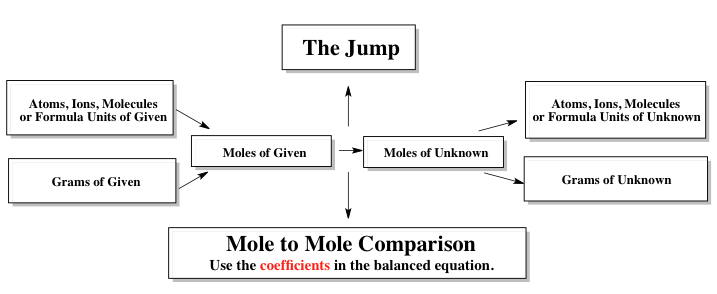Clutch Prep is now a part of Pearson
Ch.1 - Chemical MeasurementsWorksheetSee all chapters

# Volumetric Titrations

See all sections
Sections
SI Units
Metric Prefixes
Chemical Concentrations
Volumetric Analysis
Volumetric Titrations

Classical Stoichiometric Methods involve the use of both volumetric methods (titrations) and gravimetric methods.

###### Titrations

Example #1: When dealing with volumetric calculations we utilize a stoichiometric chart

###### Expert Q&A

Ask unlimited questions and get expert help right away.Example #2: Iron (III) can be oxidized by an acidic K2Cr2O7 solution according to the net ionic equation below. How many microliters of a 0.250 M FeCl2 are needed to completely react with 9.12 g of a compound containing 41.5% weight K2Cr2O7?

Cr2O72-  +  6 Fe2+  + 14 H+   →   2 Cr3+  +  6 Fe3+   +  7 H2O

###### Expert Q&A

Ask unlimited questions and get expert help right away.

###### Titrations Calculations

Example #3: Magnesium reacts with HCl according to the reaction below. How many grams of 5.310% by weight of aqueous magnesium are required to provide a 25% excess to react with 75.0 mL of 0.0550 M HCl.

Mg (s)  +   2 HCl (aq)  →  MgCl2 (aq) +  H2 (g)

###### Expert Q&A

Ask unlimited questions and get expert help right away.

Example #4: The density of 2.20 M solution of methanol (CH3OH) is 0.976 g/mL. What is the molality of the solution? The molar mass of methanol is 31.034 g/mol.

###### Expert Q&A

Ask unlimited questions and get expert help right away.

Example #5: A 0.4317 g sample of CaCO3 (MW: 100.09 g/mol) is added to flask that also contained 12.50 mL of 1.530 M HBr.

CaCO3 (aq)  +  2 HBr (aq)  →  CaBr2 (aq)  +  H2O (l)  +  CO2 (g)

Additional water is then added to create a 250.0 mL of Solution A. Next 20.00 mL aliquot of solution A is taken and titrated with 0.0980 M NaOH. How many milliliters of NaOH were used?

NaOH (aq)  +  HBr (aq)  →  H2O (l)  +  NaBr (aq)

###### Expert Q&A

Ask unlimited questions and get expert help right away.

Example #6: A 9.2476 g sample of M(OH)2 was mixed with 15.00 mL of 1.530 M HI and diluted to a final 125.0 mL of solution.

M(OH)2 (aq)  +  2 HI (aq)  →  2 H2O (l)  +  MCl2 (aq)

A 12.00 mL aliquot of this diluted solution was taken and titrated with 18.23 mL of 0.0695 M NaOH.

NaOH (aq)   +  HI (aq)  → H2O (l)  +  NaCl (aq)

What is the identity of the metal representing M?

###### Expert Q&A

Ask unlimited questions and get expert help right away.

Example #7: A 6.2034 g sample of Cu(OH)n was mixed with 25.00 mL of 2.250 M Hl and diluted to a final 250.0 mL of solution.

Cu(OH)n (s)  +  n HI (aq)  →  CuIn (aq)  +  n H2O (l)

A 50.00 mL aliquot of this solution was taken and titrated with 16.25 mL of 0.1250 M KOH. What is the value of n?

KOH (aq)  +  HI (aq)  →  H2O (l)  +  NaI (aq)

###### Expert Q&A

Ask unlimited questions and get expert help right away.

Practice: A 1.000 g sample of Na2CO3 (MW: 105.99 g/mol) is dissolved in enough water to make 200.0 mL of solution. A 25.00 mL aliquot required 32.18 mL of HCl to completely neutralize it. What is the molar concentration of HCl?

Na2CO3 (aq)  + 2 HCl (aq)  →  2 KCl (aq)  +  H2O(l)  +  CO2  (g)

###### Expert Q&A

Ask unlimited questions and get expert help right away.